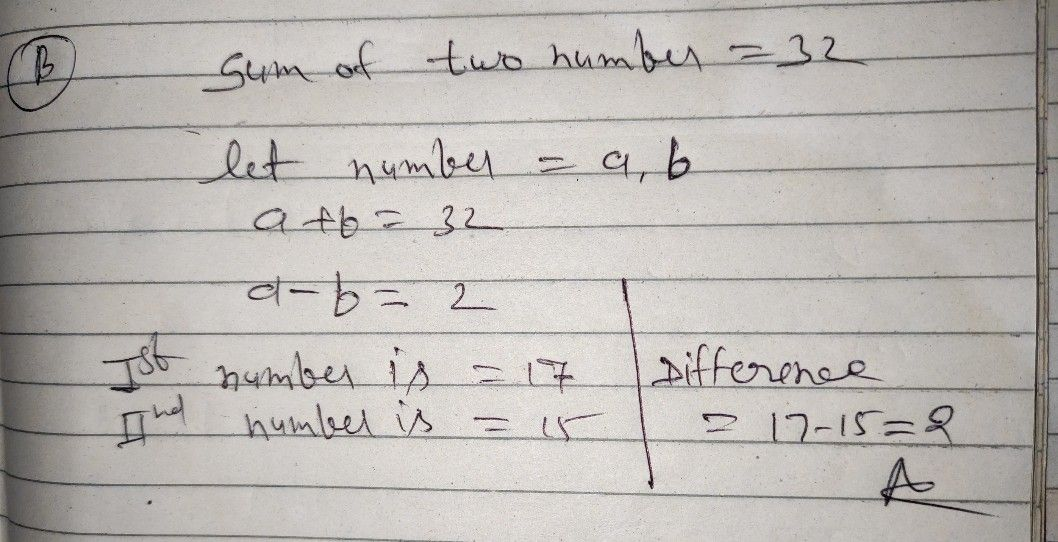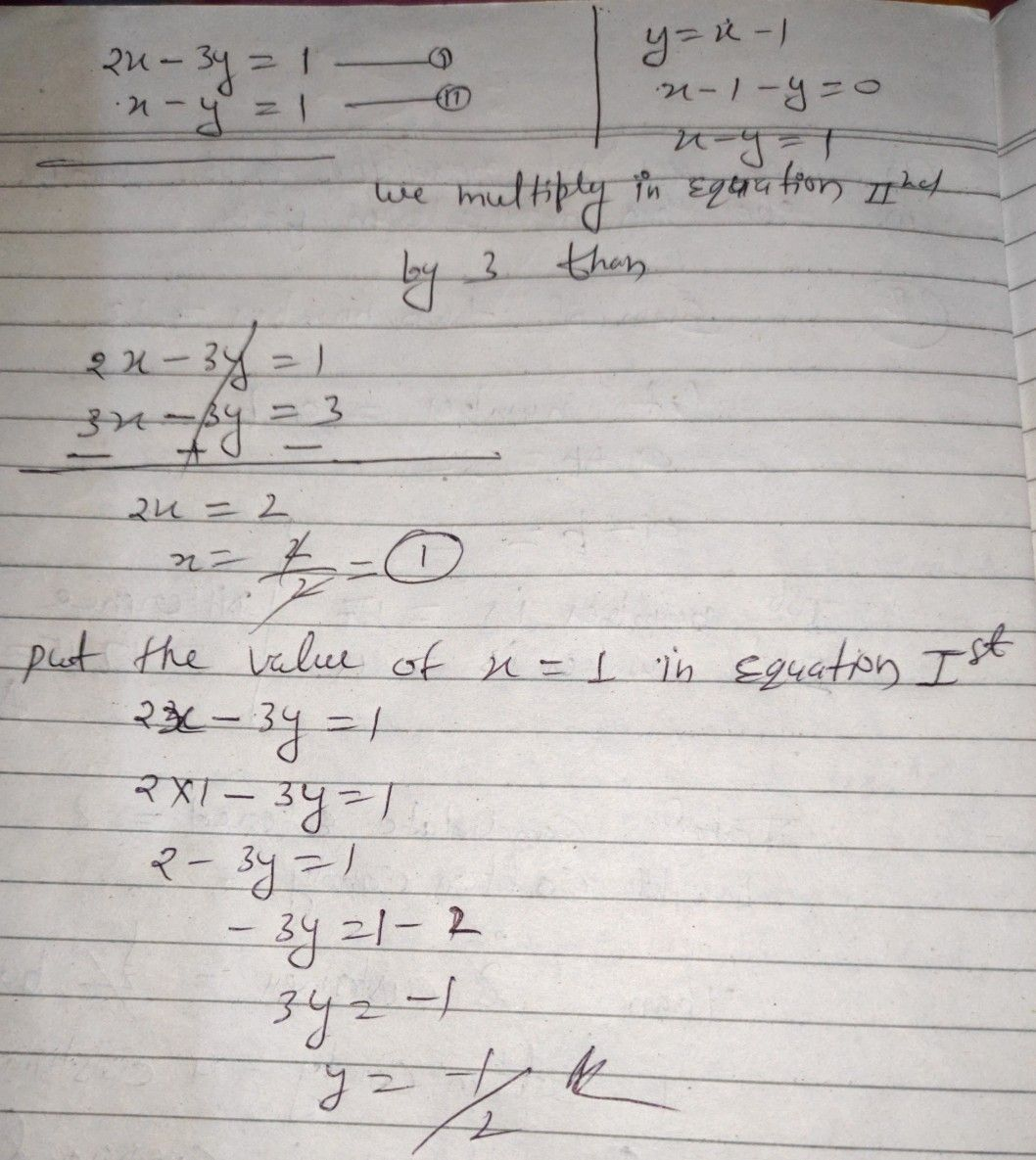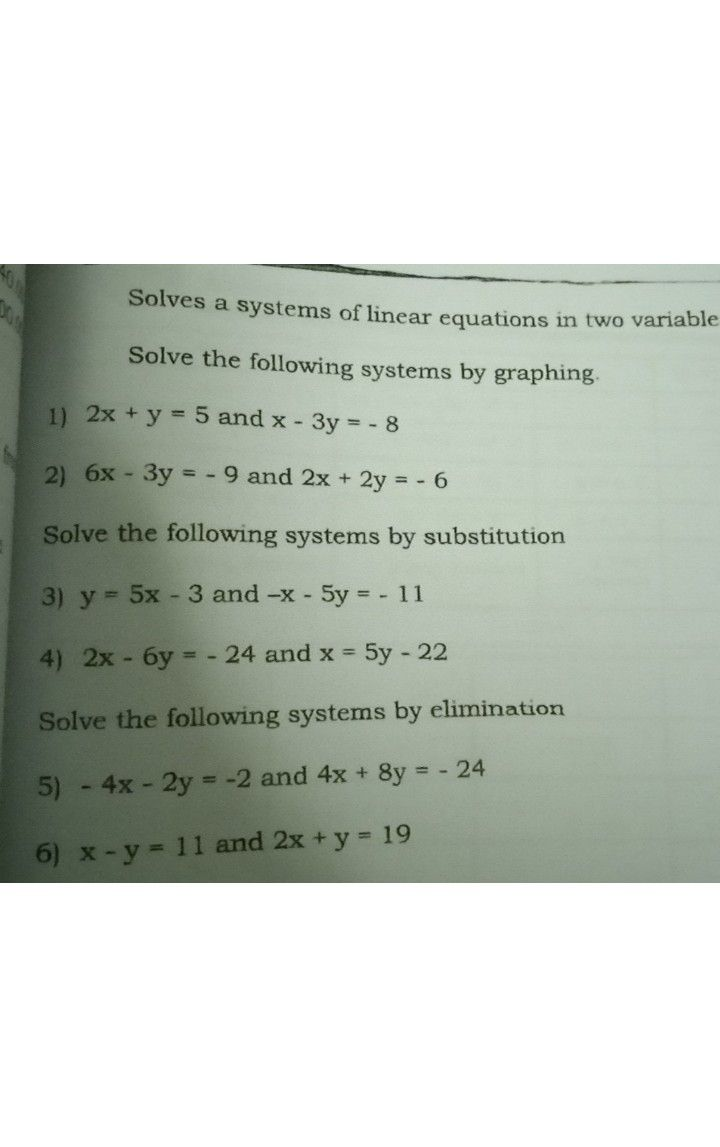Symbol
ProblemLearning Task $2$ $A$ Solve the systems $o1$ equations $by$ a) graphing b) elimination $c$ substit Eq. $1:$ $2x-3y=-1$ Eq. $a.2:y=x-1$ B. Solve the problem using any method. The sum of two numbers is $32$ and the difference $1s$ $2$ Find the numbers
Other
SolutionQanda teacher - ASHU13696Student